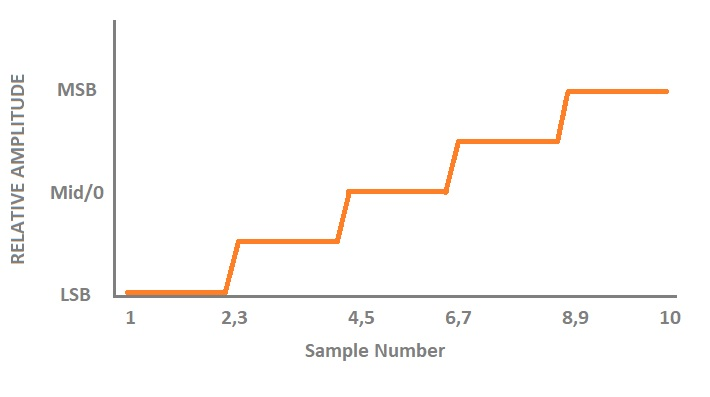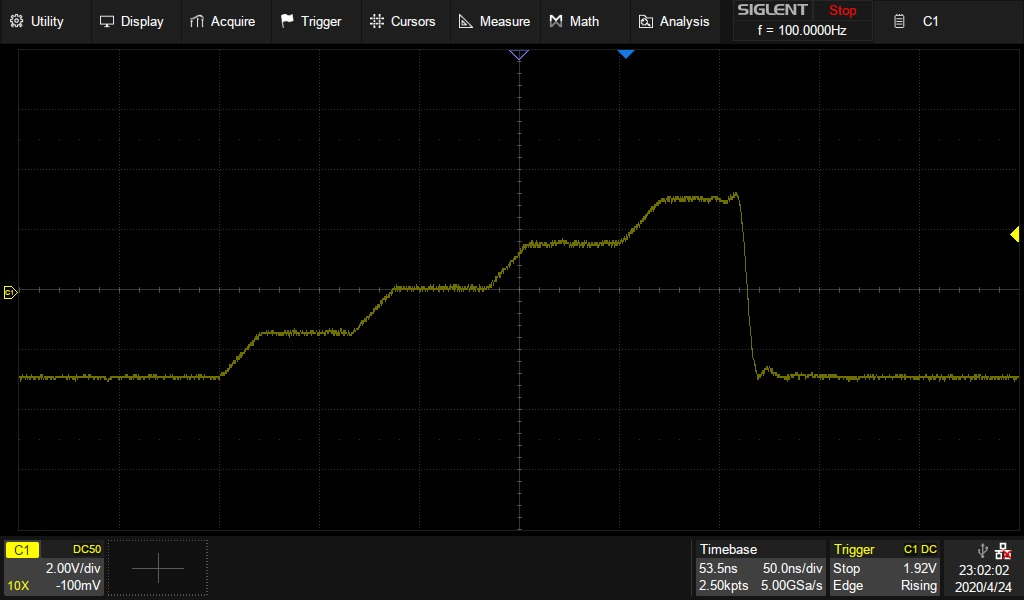# Programming Example: Create a stair-step waveform using MATLab (SDG1000X, SDG2000X, SDG6000X)

March 10, 2022

The SIGLENT SDG series of arbitrary waveform generators can use waveforms created programmatically.

The waveform data can be sent as individual samples formatted as binary little-endian, 2s complement values.

In this programming example, we create a 10 point arbitrary waveform that starts at the least-significant bit and steps up to the most-significant bit to help with understanding the required sample format as well as provide a base for your own waveform creation.

Here is a picture of the desired waveform:The SDG waveform data requires each sample to first be formatted as binary, little-endian, 2’s complement. For easier human viewing, the example enters the data in hex format and then “unhexes” the data before it is added to the command string which is then sent to the instrument.

Here is a table showing the value of each sample and the value in hex for 14-bit (SDG1000X series) and 16-bit (SDG2000X/SDG6000X) instruments:Here is an oscilloscope capture of a single burst of this waveform:# MATLAB Example

Here is a MATLAB SDG Stair Step Example